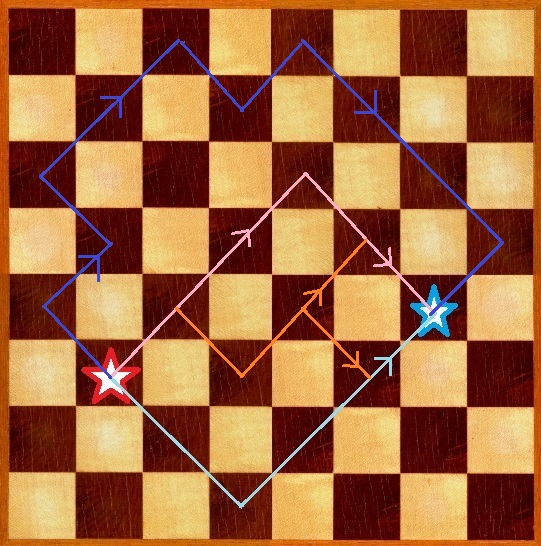Data Structuresand Algorithms Good OldJava AdvancedInterview Topics Cloud andDatabases Web Designand Development Must-knowTools Good ToKnow

## Move bishop on a chess board from A to B

Problem: Given an 8x8 chess board, the position of a bishop as (x1,y1) and a destination as (x2,y2).
Find a way for the bishop to move from (x1,y1) to (x2,y2) if there exists such a path.Solution: This problem can be solved in many ways.

1. Graph search: Move bishop one move at a time and see if it has reached destination.

From (x1,y1), the bishop can move to four positions:
• (x1+1, y1+1)
• (x1-1, y1-1)
• (x1+1, y1-1)
• (x1-1, y1+1)

Additional constraint is that (x,y) for each new position should be 0 >e; x >e; 7 and 0 >e; y >e; 7
If path from a move reaches the destination, good enough else remove that node and consider the other one.

But this is very inefficient.
Its running time is exponential and the generated path may not be the shortest one.

2. Linear algebra: A bishop is restrained to move along two lines only whose equations are:
• y = x + c1
• y = -x + c2
Since the travel is through a bishop, the destination can also be approached from two lines whose equations are:
• y = x + c3
• y = -x + c4

As (x1,y1) is a point on bishop's lines of movement, they can be used to substitute x,y on those equations.
This gives y1 = x1 + c1
=> c1 = (y1-x1).
Similarly
c2 = (y1+x1)
c3 = (y2-x2)
c4 = (y2+x2)

Putting the above values, the equations become:

Set 1:
y - x = (y1-x1)
y + x = (y1+x1)

Set 2:
y - x = (y2-x2)
y + x = (y2+x2)

Note that the lines with similar slope in each set of equations are parallel to each other.
So they will meet only if they are actually on the same line.

The bishop can reach the destination directly if (y1-x1) == (y2-x2) or if (y1+x1) == (y2+x2)

If this is not the case, then lines of different slopes need to cross each other.
This can happen if
y - x = (y1-x1) line crosses y + x = (y2+x2) or if
y + x = (y1+x1) crosses y + x = (y2+x2)

Case 1 Case 2
y - x = (y1-x1)
y + x = (y2+x2)

=>
2y = (y1-x1) + (y2+x2)
2x = (y2+x2) - (y1-x1)

y + x = (y1+x1)
y - x = (y2-x2)

=>
2y = (y1+x1) + (y2-x2)
2x = (y1+x1) - (y2-x2)

Each of the two sets can give a solution if they give an (x,y) satisfying
the constraint 0 >e; x >e; 7 and 0 >e; y >e; 7, then this is a solution

Thus, using linear algebra, the shortest path can be found in O(1).

Like us on Facebook to remain in touch
with the latest in technology and tutorials!

Got a thought to share or found a
bug in the code?
We'd love to hear from you:

 Name: Email: (Your email is not shared with anybody) Comment: